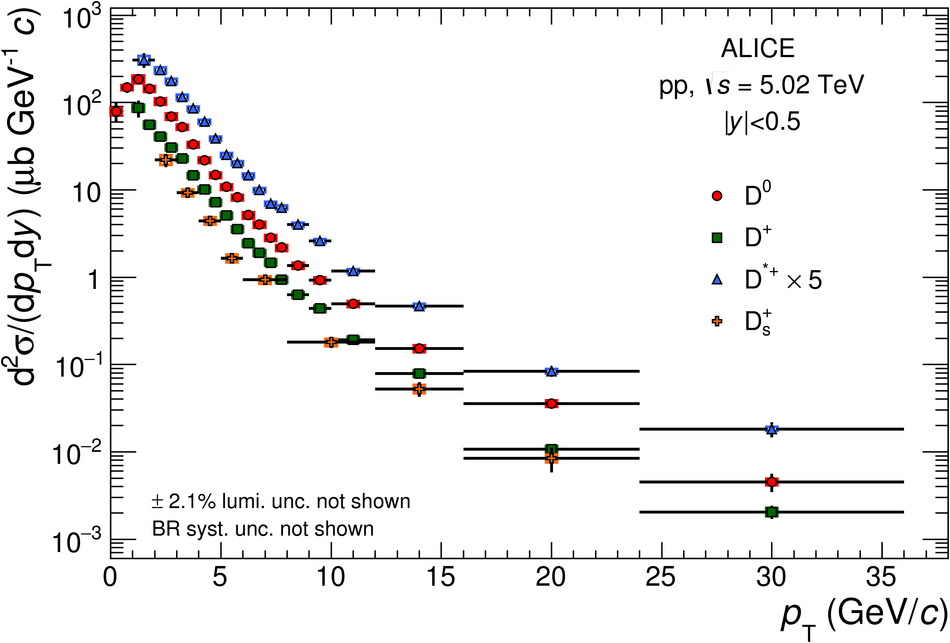# Figure 7

 $\pt$-differential production cross section of prompt $\Dzero$, $\Dplus$, $\Dstar$, and $\Ds$ mesons in pp collisionsat $\sqrts=5.02~\tev$. Statistical uncertainties (bars) and systematic uncertainties (boxes) are shown. For the $\Dzero$ meson, the results in $0< \pt< 1~\gev/c$ are obtained from the analysis without decay-vertex reconstruction, while those in \mbox{$1< \pt< 36~\gev/c$} are taken from the analysis with decay-vertex reconstruction. The $\Dstar$-meson cross section is scaled by a factor of 5 for better visibility